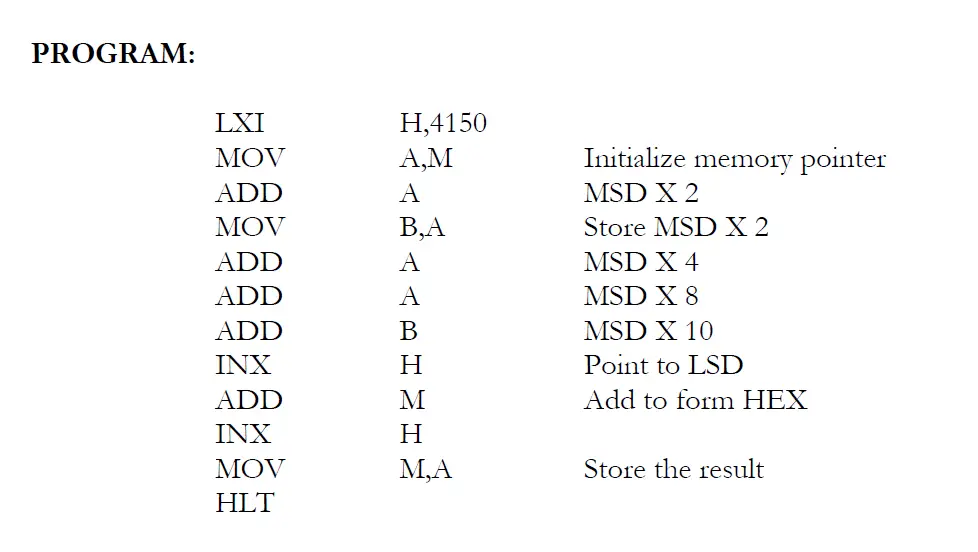# BCD to HEX Conversion program in 8085 – PhysicsTeacher.in

Last updated on March 10th, 2022 at 03:34 pm

In this post, we will see how To convert two-digit packed BCD numbers in memory to the equivalent HEX number using the 8085 microprocessor program or instruction set.

## Algorithm for BCD to HEX Conversion with 8085 microprocessor

1) Initialize memory pointer to 4150 H
2) Get the Most Significant Digit (MSD)
3) Multiply the MSD by ten using repeated addition
4) Add the Least Significant Digit (LSD) to the result obtained in the previous step
5) Store the HEX data in Memory

## Program to convert two digit packed BCD numbers to HEX in 8085

LXI H,4150
MOV A,M Initialize memory pointer
MOV B,A Store MSD X 2
INX H Point to LSD
INX H
MOV M,A Store the result
HLTProgram to convert two BCD numbers in memory to the equivalent HEX number using the 8085 microprocessor (BCD to HEX Conversion)

## Observation

Input: 4150 : 02 (MSD)
4151 : 09 (LSD)
Output: 4152 : 1D H

## Result

Thus the 8085 program to convert BCD data to HEX data was executed.